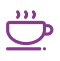Home / Coffee /
Unveiling the Beauty of Fractal Patterns in Nature

# Unveiling the Beauty of Fractal Patterns in Nature

By Lorenzo Sevilla
Apr 14, 2023

Fractal patterns have fascinated mathematicians, scientists, and artists alike for centuries. These complex and intricate patterns can be found throughout the natural world, from the branching of trees to the shape of snowflakes. In this article, we will delve into the world of fractal patterns, exploring what they are, where they can be found, and why they are so fascinating.

## What are fractal patterns?

Fractal patterns are mathematical constructs that exhibit self-similarity at different scales. In other words, a fractal pattern looks the same when viewed up close or from far away. Fractals are generated by repeating a simple process or equation over and over again. The resulting pattern is a fractal, which is often infinitely complex.

What are examples of fractals in nature? Fractal patterns can be found all around us in the natural world. One of the most well-known examples is the branching patterns of trees, which exhibit self-similarity as the branches split into smaller and smaller branches. Other examples include the shape of snowflakes, the coastline of a continent, and the structure of a lightning bolt. Fractal patterns can even be found in the human body, such as the branching of blood vessels and the structure of the lungs.

## What are the 4 types of fractals?

There are four main types of fractals: Iterated Function Systems (IFS), Strange Attractors, Fractal Landscapes, and L-Systems. IFS fractals are created by repeatedly applying a set of functions to a starting shape. Strange attractors are generated by iterating a set of equations that describe the behavior of a chaotic system. Fractal landscapes are created using algorithms that simulate the erosion and formation of natural features such as mountains and rivers. L-Systems are used to generate branching patterns such as the ones found in trees.

## What are fractal patterns in the natural world?

Fractal patterns are found throughout the natural world, from the microscopic to the macroscopic scale. In addition to the examples listed above, fractal patterns can be seen in the shape of seashells, the patterns on a butterfly's wings, and the structure of fern leaves. Fractal patterns are also present in the way that clouds form and the shape of lightning bolts.

## What are fractals?

Fractals are mathematical constructs that exhibit self-similarity at different scales. They are generated by repeating a simple process or equation over and over again. Fractal patterns are infinitely complex and can be found throughout the natural world.

Fractal pattern examples Fractal patterns can be found in many natural phenomena, such as the branching of trees, the shape of snowflakes, and the patterns on a butterfly's wings. Fractal patterns can also be seen in the structure of seashells, the way that clouds form, and the shape of lightning bolts.

## What is a fractal pattern?

A fractal pattern is a mathematical construct that exhibits self-similarity at different scales. Fractal patterns are generated by repeating a simple process or equation over and over again. The resulting pattern is infinitely complex and can be found throughout the natural world.

## Fibonacci fractals in nature

The Fibonacci sequence is a mathematical sequence in which each number is the sum of the two preceding numbers. This sequence is found throughout the natural world, from the branching of trees to the pattern of seeds in a sunflower. Fibonacci fractals are generated using the Fibonacci sequence to create branching patterns similar to those found in trees and other natural structures.

Fractals can be found at every scale, from the smallest cellular structures to the largest astronomical bodies. The self-similar nature of fractals creates patterns that are both intricate and beautiful, inspiring artists and scientists alike.

One example of a fractal in nature is the meanders pattern found in rivers. Meanders are created by the flow of water eroding the outside of a bend and depositing sediment on the inside of the bend. As the river continues to flow, the meanders become more complex and self-similar, creating a fractal pattern. Another example is the shape of seashells, which exhibit a logarithmic spiral pattern. This spiral is created by the growth of the shell, with each successive spiral being slightly larger and self-similar to the previous one.

Flower fractals are another example of the presence of fractals in nature. The structure of flowers, such as the head of a sunflower or the arrangement of petals on a daisy, follows a pattern of self-similarity that can be described mathematically. The Fibonacci sequence, mentioned earlier, is often used to explain the pattern of flower petals and the arrangement of leaves on a stem.

## Conclusion

In conclusion, fractal patterns are a fascinating and beautiful aspect of the natural world. These complex and infinitely intricate patterns can be found at every scale, from the smallest cellular structures to the largest astronomical bodies. Fractals are generated by repeating a simple process or equation over and over again, resulting in patterns that exhibit self-similarity at different scales. From the branching of trees to the shape of snowflakes, fractals can be found all around us, inspiring artists, mathematicians, and scientists to explore their hidden mathematical wonders.

#### CategoriesCoffee